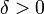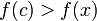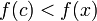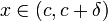First derivative test

Statement

What the test is for

The first derivative test is a partial (i.e., not always conclusive) test used to determine whether a particular critical point in the domain of a function is a point where the function attains a local maximum value, local minimum value, or neither. There are cases where the test is inconclusive, which means that we cannot draw any conclusion.

What the test says: one-sided sign versions

Suppose$f$ is a function defined at a point$c$.

Then, we have the following:

Continuity and differentiability assumption Hypothesis on sign of derivative Conclusion$f$ is left continuous at$c$ and differentiable on the immediate left of$c$$\! f'(x)$ is positive (respectively, nonnegative) for$x$ to the immediate left of$c$ (i.e., for$x \in (c - \delta, c)$ for sufficiently small$\delta > 0$)$f$ has a strict local maximum from the left at$c$, i.e.,$f(c) > f(x)$ (respectively,$f$ has a local maximum from the left at$c$, i.e.,$f(c) \ge f(x)$) for$x$ to the immediate left of$c$.$f$ is left continuous at$c$ and differentiable on the immediate left of$c$$\! f'(x)$ is negative (respectively, nonpositive) for$x$ to the immediate left of$c$ (i.e., for$x \in (c - \delta, c)$ for sufficiently small$\delta > 0$)$f$ has a strict local minimum from the left at$c$, i.e.,$f(c) < f(x)$ (respectively,$f$ has a local minimum from the left at$c$, i.e.,$f(c) \le f(x)$) for$x$ to the immediate left of$c$.$f$ is right continuous at$c$ and differentiable on the immediate right of$c$$\! f'(x)$ is positive (respectively, nonnegative) for$x$ to the immediate right of$c$ (i.e., for$x \in (c,c + \delta)$ for sufficiently small$\delta > 0$)$f$ has a strict local minimum from the right at$c$, i.e.,$f(c) < f(x)$ (respectively,$f$ has a local minimum from the right at$c$, i.e.,$f(c) \le f(x)$) for$x$ to the immediate right of$c$.$f$ is right continuous at$c$ and differentiable on the immediate right of$c$$\! f'(x)$ is negative (respectively, nonpositive) for$x$ to the immediate right of$c$ (i.e., for$x \in (c,c + \delta)$ for sufficiently small$\delta > 0$)$f$ has a strict local maximum from the right at$c$, i.e.,$f(c) > f(x)$ (respectively,$f$ has a local maximum from the right at$c$, i.e.,$f(c) \ge f(x)$) for$x$ to the immediate right of$c$.

What the test says: combined sign versions

Suppose$f$ is a function defined around a point$c$ (i.e.,$f$ is defined in an open interval containing$c$) and is continuous at$c$. We do not care whether$f$ is differentiable at$c$; however, the test makes sense only if$f$ is differentiable on the immediate left and immediate right of$c$.

Then, we have the following (we list only the strict cases in the table below):

Continuity and differentiability assumption Sign of the derivative$f'$ on immediate left of$c$ Sign of$f'$ on immediate right of$c$ Conclusion about local minimum, local maximum, or neither$f$ is continuous at$c$ and differentiable on the immediate left and immediate right of$c$ positive negative strict local maximum$f$ is continuous at$c$ and differentiable on the immediate left and immediate right of$c$ negative positive strict local minimum$f$ is continuous at$c$ and differentiable on the immediate left and immediate right of$c$ positive positive neither local maximum nor local minimum, because$f$ is increasing through the point$f$ is continuous at$c$ and differentiable on the immediate left and immediate right of$c$ negative negative neither local maximum nor local minimum, because$f$ is decreasing through the point

If we replace positive by nonnegative and negative by nonpositive in the rows corresponding to strict local maximum and strict local minimum, we could potentially lose the strictness.

Note that if$f'$ has ambiguous sign on the immediate left or on the immediate right of$c$, the first derivative test is inconclusive.

Relation with critical points

The typical goal of the first derivative test is to determine whether a critical point is a point of local maximum or minimum. Hence, the test is typically applied to critical points. However, when applying the first derivative test, we do not need to check whether the point in question is a critical point. In other words, if the condition for being a point of local maximum or minimum is satisfied, then the point in question is automatically a critical point and this condition need not be checked separately.

Succinct version

Here is a shorter version: at a critical point, if the derivative changes sign from negative to positive (as we go from left to right) then that is a point of local minimum. If the derivative changes sign from positive to negative (as we go from left to right) then it is a point of local maximum.